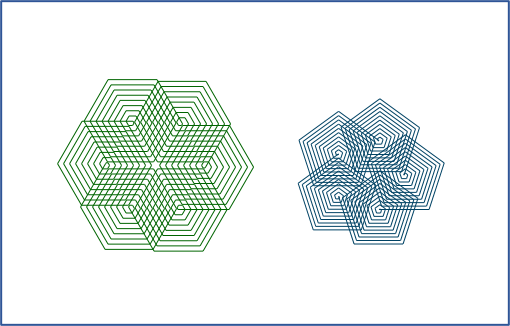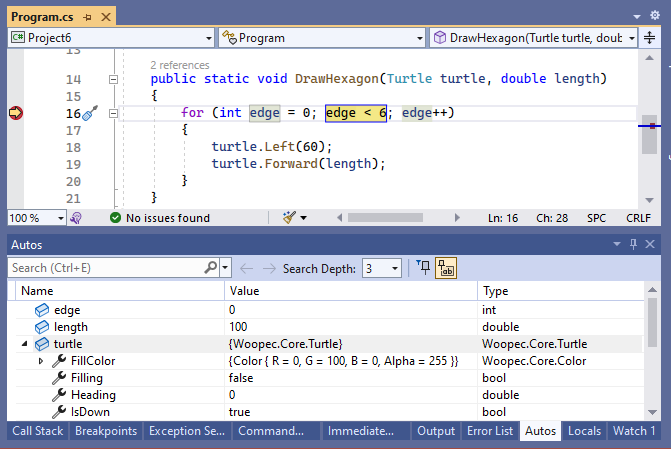It is not enough for your code to do what it is supposed to do. You should strive to write clean code from the start. Even as a beginner, you can use code conventions and follow the DRY principle. This will help you write code that is easier to understand and extend. In this post, we use C# variables and loops to improve code that draws a hexagon.

### Beginner code for drawing a hexagon

``````using Woopec.Core;

internal class Program
{
public static void WoopecMain()
{
var pen = Pen.CreateExample();
pen.IsDown = true;

pen.Rotate(60);
pen.Move(100);
pen.Rotate(60);
pen.Move(100);
pen.Rotate(60);
pen.Move(100);
pen.Rotate(60);
pen.Move(100);
pen.Rotate(60);
pen.Move(100);
}
}
``````

The `Pen` class follows similar concepts as the`Turtle` class from this previous article:

• First, a simple pen is created.
• If the pen should draw during its movement, its `IsDown` property must be set to `true`.
• The Pen always draws in a certain direction. For changing this direction there is the method `Rotate`. A value greater than 0 rotates to the left and a value less than 0 rotates to the right.
• The `Move` method changes the position of the pen. A positive value moves the pen forward, a negative value moves it backward.
• As an alternative to `Rotate` and `Move` you can also use the pen method `SetPosition` for drawing. But for now we stay with Rotate and Move.

### Clean Code

The above code draws a hexagon. So it does exactly what it is supposed to do. But that is not the only criterion for good code. Code should also be easy to understand when you read it. And code should be easy to change. There is a book on this called “Clean Code. A Handbook of Agile Software Craftsmanship” by Robert C. Martin. It starts with this sentence:

Writing clean code is what you must do in order to call yourself a professional. There is no reasonable excuse for doing anything less than your best.

It talks about professionals here, but from my point of view, it applies just as much to beginners. You should write as clean code as possible from the beginning. This will help you to make much better progress in the end.

Let’s see how we can write the above code more cleanly. At the same time we will learn some new C# constructs.

### Variables

What would we have to do if we wanted the edges in our program to be only 50 pixels long instead of 100 pixels?

We would have to replace the `100` with a `50` in six places. This is quite cumbersome. It is much easier if we define and use a C# variable. We can define a variable like this:

``````    var edgeLength = 50;
``````

The `var` keyword tells the C# compiler that a variable should be defined here. The text `edgeLength` is the variable name, here we can think of any name we want. But the name may only consist of letters, numbers and a few special characters (e.g. a `_`), spaces are not allowed. After the equal sign there follows the value of the variable, here `50`. This variable can now be used wherever the edge length is needed. We can change the relevant part of the program like this:

``````    var edgeLength = 50;

pen.Rotate(60);
pen.Move(edgeLength);
pen.Rotate(60);
pen.Move(edgeLength);
pen.Rotate(60);
// ...
``````

### Coding Conventions

In principle you can name a variable as you like. However, programming is easier if you follow a few rules, so-called Coding Conventions. For the names of variables there are these conventions:

• It should be recognizable from the name what it is about. Therefore the name `edgeLength` is better than a name that, for example, consists of only one letter.
• Variable names start with a lowercase letter. This makes it easier to distinguish variables from other things.
• If the name consists of several words, the other words each start with a capital letter. This spelling is also called camelCase.

Further information about naming conventions can be found for example at Wikipedia or at Microsoft.

### The DRY-Principle: Don’t Repeat Yourself

In our hexagon program, these lines are repeated six times:

``````    pen.Rotate(60);
pen.Move(edgeLength);
``````

This is bad for several reasons: if we want to change something, for example replace `100` with `edgeLength`, we have to change it in several places. This is superfluous work. And if we forget one place when changing, our program will not work as it should.

So we should change our program in a way that we don’t have to repeat ourselves in the code.

For this we need…

### Loops

For doing the same thing multiple times, C# has loops. For example, we can change our program to use a while loop:

``````    var edgeCounter = 0;
while (edgeCounter < 6)
{
pen.Rotate(60);
pen.Move(edgeLength);
edgeCounter = edgeCounter + 1;
}
``````

Here we have used several C# constructs:

• First we define a new variable `edgeCounter`. With this variable we count how many edges have already been drawn.
• Whenever we have drawn an edge, we increase the value of `edgeCounter` by adding one. It works like this: `edgeCounter = edgeCounter + 1;`
• We have to repeat this as long as we have drawn less than six edges. We specify it like this: `while (edgeCounter < 6)` The character `<` stands for `is less than`. So by `edgeCounter < 6` we check if the value of the variable `edgeCounter` is smaller than `6`.
• This is followed by an opening curly bracket `{` and further down by a closing bracket `}`. These two brackets define a so-called code block. All lines of code between these two brackets belong to this code block and will be executed multiple times.
• To be able to recognize where the code block starts and where it ends, the brackets are written in separate lines and the content of the code block is indented by four characters (you can also press the Tab key). We do this kind of code indentation uniformly throughout the code so that we can easily recognize code blocks everywhere. This is also a coding convention.

Loops can be written even more compactly as a for-loop:

``````    for (var edgeCounter = 0; edgeCounter < 6;  edgeCounter++)
{
pen.Rotate(60);
pen.Move(edgeLength);
}
``````

The `for` loop uses the same elements as the while loop, but here they are on a single line: First the `edgeCounter` variable is defined, then a semicolon follows, then a condition specifies how often the loop should be executed, then another semicolon follows, and at the end there is the statement to increment the variable by one. Because it often happens that you want to increment a variable by one, there is also a special statement for this. Instead of `edgeCounter = edgeCounter + 1` you can simply write `edgeCounter++`.

There are also other forms of loops. And there are also many other possibilities when defining conditions. I don’t explain these in more detail here, because there is a good explanation page from Microsoft. You should have a look at all the examples on this page and try them out: C# if statements and loops - conditional logic tutorial.

### DRY principle, part 2: Simplifying the code even further

Our code is much cleaner now. But it does not yet follow the DRY principle one hundred percent.

Where does the number `60` in `seymour.Rigth(60)` come from? This number has to do with the number of edges: Because we want to draw a regular 6-corner, the pen must rotate by `360.0 / 6` degrees. So actually the number `6` appears twice: Once in the for condition (`edgeCounter < 6`). And a second time in the calculation of the rotation (`360 / 6`). We can also improve this: We define and use a variable `numberOfEdges` that specifies the desired number of edges. Then we can change our program like this:

``````public static void WoopecMain()
{
var pen = Pen.CreateExample();
pen.IsDown = true;

var edgeLength = 50;
var numberOfEdges = 6;

for (var edgeCounter = 0; edgeCounter < numberOfEdges; edgeCounter++)
{
pen.Rotate(360.0 / numberOfEdges);
pen.Move(edgeLength);
}
}
``````

Compared to the initial version, the code has become shorter, there are no repetitions, and the variable names explain the meaning of values. All in all, the code is much easier to understand.

There is another advantage: What would we have had to change in the initial code if we had wanted to draw a 17-corner? We would have had to add many more statements, and we would have had to replace the number 60 with another number. In the current version of the code, we only need to change one place. We change the definition of numberOfEdges to 17:

``````    var numberOfEdges = 17;
``````

This single change causes the code to draw a 17-corner instead of a 6-corner.

From this example, you can see how important it is to write clean code.

### Debugging of variables

When using variables, errors can happen. But also here the debugger helps. If you put the cursor on the check `edgeCounter < numberOfEdges`, you can create a breakpoint there with F9 (see previous article). When you then start the program in the debugger (with F5), it stops at this breakpoint. You can then see in the debugger what values the variables have:Below the code window, Visual Studio displays an Autos window. This window displays the values of the variables that are currently playing a role. You can see in this example that `edgeCounter` has the value 0 and `numberOfEdges` has the value 6. You can then run the code step by step (with F10) or until the next breakpoint (with F5) and always see the current value of the variable.

The debugger has many more features for variables. For example, moving the mouse cursor over a variable in the code will automatically display its value. You can find more information about debugging variables in the Microsoft documentation.

### Summary

This post is part of a series. You can find the previous post here, an overview here and the next post here.

Clean Code

• Follow coding conventions for indentation and variable names, see Microsoft Naming Conventions.
• Don’t repeat yourself (DRY principle).

C#

• Variable definition (`var edgeCounter = 0`), change (`edgeCounter = edgeCounter + 1;`), check (`edgeCounter < 6`) and usage (`Right(360-0 / edgeNumber`).
• Loops (`for` or `while`), see Microsoft Tutorial.
• Definition of conditions, for example `(edgeCounter < 6)`. There are many more possibilities, see Microsoft Tutorial.

Debugging

Woopec library

• Advanced users can use the Pen class instead of the Turtle class.
• In this article the methods `Rotate` and `Move`, as well as the property `IsDown` were used. For more information, see the Woopec documentation.

### How to go on

For example, you could try the following: Change the code to use a `while` loop again instead of a `for` loop. Then change the code to decrease the edge length by one in each loop pass. And then change the condition for the loop so that the loop is not terminated until the edge length has the value 1. What is the result?